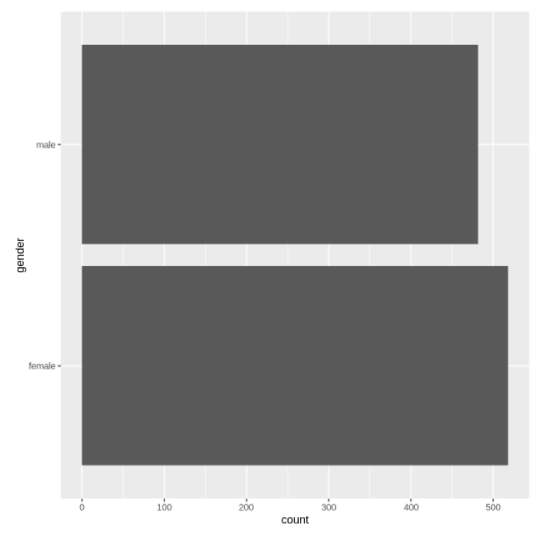# Tutorial for Bar Plot in ggplot2 with Examples## Syntax of Bar Plot in ggplot2

In ggplot2, the following is the minimal syntax for generating the bar plot  –

ggplot(<data>, mapping = aes(<mapping>) + geom_bar()

You can customize the bar plot by adding more layers such as themes, labs, etc.

The ggplot2 library can be loaded with the below line.
In :
`library(ggplot2)`

In the below example, we are plotting the very basic bar plot in ggplot2 by passing the dataset along with the mapping of attributes for x and y.

### Example 2: Horizontal Bar PlotOut:
Out:
Out: